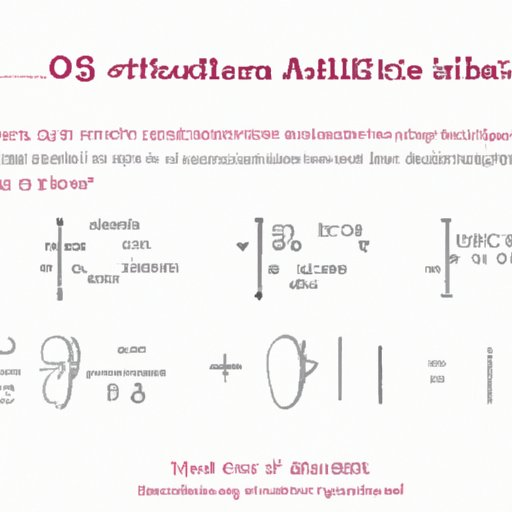# What Math is Needed for Artificial Intelligence? An Overview of the Types of Mathematics Used## Introduction

Artificial Intelligence (AI) has become an essential part of our daily lives, from smart phones and self-driving cars to medical diagnostics and digital assistants. But what math is needed for AI to function? This article will explore the types of mathematics needed for AI, and how they are applied to AI algorithms.

## Definition of Artificial Intelligence

Artificial Intelligence (AI) is a branch of computer science that studies how to create machines that can think and learn like humans. AI systems use algorithms to process data, recognize patterns, and make decisions. AI is used in many different fields, such as robotics, medicine, finance, and marketing.

## Overview of Types of Math Needed for AI

The types of math needed for AI include Calculus, Linear Algebra, Discrete Mathematics, Probability and Statistics. Each type of math is necessary for AI to function properly.

## Exploring the Types of Math Needed for AI

Calculus is used to analyze functions and optimize them. Calculus is used to model decision making processes and develop strategies for AI systems. It is also used to calculate derivatives and integrals, which are essential for machine learning algorithms.

Linear Algebra is used to represent vectors, matrices, and linear equations. Linear Algebra is used to analyze data and determine relationships between variables. It is also used to solve optimization problems, which are essential for AI algorithms.

Discrete Mathematics is used to study the properties of discrete objects. Discrete Mathematics is used to design algorithms and analyze their complexity. It is also used to understand the behavior of algorithms, which is essential for AI systems.

Probability and Statistics are used to study the behavior of random variables. Probability and Statistics are used to analyze data and make predictions about future events. They are also used to evaluate the performance of AI algorithms.

## Understanding Mathematical Foundations of AI

Bayesian Networks are used to represent probabilistic relationships between variables. Bayesian Networks are used to infer the probability of an event given certain conditions. They are also used to predict outcomes based on past data, which is essential for AI applications.

Information Theory is used to measure the amount of information contained in a system. Information Theory is used to create models that can accurately represent data. It is also used to assess the accuracy of AI algorithms.

Graph Theory is used to represent networks of interconnected nodes. Graph Theory is used to analyze complex systems, such as social networks or the internet. It is also used to create AI algorithms that can navigate these networks.

## Applying Mathematics to AI Algorithms

Neural Networks are used to approximate nonlinear functions. Neural Networks are used to classify data and make predictions. They are also used to identify patterns in data, which is essential for AI applications.

Decision Trees are used to represent decision-making processes. Decision Trees are used to classify data and make decisions. They are also used to identify patterns in data, which is essential for AI applications.

Support Vector Machines are used to classify data and make predictions. Support Vector Machines are used to find the optimal solution to a problem. They are also used to identify patterns in data, which is essential for AI applications.

## Examining the Role of Probability in AI

Conditional Probability is used to measure the probability of an event occurring given certain conditions. Conditional Probability is used to make predictions about future events. It is also used to assess the accuracy of AI algorithms.

Bayes Theorem is used to calculate the probability of an event occurring given certain conditions. Bayes Theorem is used to make decisions based on uncertain information. It is also used to assess the performance of AI algorithms.

Markov Chains are used to model sequences of events. Markov Chains are used to predict future events based on past information. They are also used to assess the accuracy of AI algorithms.

## Investigating the Use of Statistics in AI

Data Collection is used to gather information about a system. Data Collection is used to analyze data and make inferences about the system. It is also used to assess the performance of AI algorithms.

Descriptive Statistics is used to summarize data. Descriptive Statistics is used to analyze data and make predictions about future events. It is also used to assess the accuracy of AI algorithms.

Inferential Statistics is used to draw conclusions from data. Inferential Statistics is used to make decisions based on uncertain information. It is also used to assess the performance of AI algorithms.

## Conclusion

Mathematics is essential for AI to function properly. Different types of math are needed for AI, from calculus and linear algebra to probability and statistics. Mathematical foundations, such as Bayesian networks and graph theory, are also necessary for AI algorithms to work. Additionally, probability and statistics play an important role in AI, from data collection to inferential statistics. In conclusion, mathematics is an integral part of AI.#### By Happy Sharer

Hi, I'm Happy Sharer and I love sharing interesting and useful knowledge with others. I have a passion for learning and enjoy explaining complex concepts in a simple way.